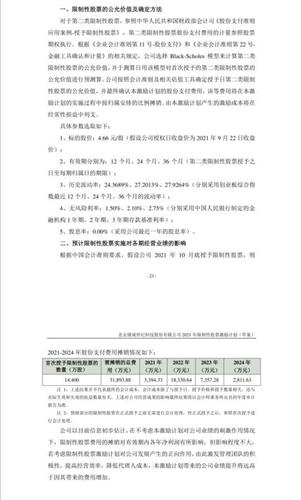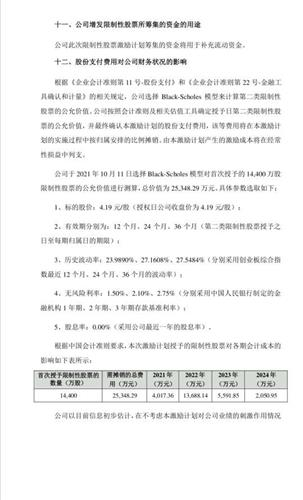N1:=20;
N2:=10;
N3:=5;
AA:=REF(H,N1)=HHV(H,2*N1+1);
QY:=BACKSET(AA,N1+1);
CC:=FILTER(QY,N1) AND H=HHV(H,N1+1);
DDD:=BARSLAST(CC);
GDTS:=IF(CC,CURRBARSCOUNT,0);
DINGBU:=IF(DDD,REF(H,DDD),H);{顶部}
DINGBUTS:=IF(DDD,REF(GDTS,DDD),GDTS);
DINGBU1:=REF(DINGBU,DDD+1);
GDCS1:=CONST(DINGBUTS);
DINGBUTS1:=REF(DINGBUTS,DDD+1);
GDCS2:=CONST(DINGBUTS1);
DINGBU2:=REF(DINGBU1,DDD+1);
MM:=IF(CURRBARSCOUNT>GDCS2,0,1);
{DRAWLINE(CURRBARSCOUNT=GDCS2,H,ISLASTBAR,REF(H,GDCS2-1),1),COLOR0055FF,POINTDOT,LINETHICK2;
DRAWLINE(CURRBARSCOUNT=GDCS1,H,ISLASTBAR,REF(H,GDCS1-1),1),COLORYELLOW,POINTDOT,LINETHICK2;}
AA1:=REF(L,N1)=LLV(L,2*N1+1);
QY1:=BACKSET(AA1,N1+1);
CC1:=FILTER(QY1,N1) AND L=LLV(L,N1+1);
DD1:=BARSLAST(CC1);
DDTS:=IF(CC1,CURRBARSCOUNT,0);
YDB:=IF(DD1,REF(L,DD1),L);
DDTS1:=IF(DD1,REF(DDTS,DD1),DDTS);
DDCS1:=CONST(DDTS1);
ZDB:=REF(YDB,DD1+1);
DDTS2:=REF(DDTS1,DD1+1);
DDCS2:=CONST(DDTS2);
{DRAWLINE(CURRBARSCOUNT=DDCS2,L,ISLASTBAR,REF(L,DDCS2-1),1),COLOR0055FF,POINTDOT,LINETHICK2;
DRAWLINE(CURRBARSCOUNT=DDCS1,L,ISLASTBAR,REF(L,DDCS1-1),1),COLORYELLOW,POINTDOT,LINETHICK2;}
LAA:=REF(H,N2)=HHV(H,2*N2+1);
LQY:=BACKSET(LAA,N2+1);
LCC:=FILTER(LQY,N2) AND H=HHV(H,N2+1);
LDDD:=BARSLAST(LCC);
LGDTS:=IF(LCC,CURRBARSCOUNT,0);
LDINGBU:=IF(LDDD,REF(H,LDDD),H);{顶部}
LDINGBUTS:=IF(LDDD,REF(LGDTS,LDDD),LGDTS);
LDINGBU1:=REF(LDINGBU,LDDD+1);
LGDCS1:=CONST(LDINGBUTS);
LDINGBUTS1:=REF(LDINGBUTS,LDDD+1);
LGDCS2:=CONST(LDINGBUTS1);
LDINGBU2:=REF(LDINGBU1,LDDD+1);
LMM:=IF(CURRBARSCOUNT>LGDCS2,0,1);
{DRAWLINE(CURRBARSCOUNT=LGDCS2,H,ISLASTBAR,REF(H,LGDCS2-1),1),COLOR0055FF,POINTDOT;
DRAWLINE(CURRBARSCOUNT=LGDCS1,H,ISLASTBAR,REF(H,LGDCS1-1),1),COLORYELLOW,POINTDOT;}
LAA1:=REF(L,N2)=LLV(L,2*N2+1);
LQY1:=BACKSET(LAA1,N2+1);
LCC1:=FILTER(LQY1,N2) AND L=LLV(L,N2+1);
LDD1:=BARSLAST(LCC1);
LDDTS:=IF(LCC1,CURRBARSCOUNT,0);
LYDB:=IF(LDD1,REF(L,LDD1),L);
LDDTS1:=IF(LDD1,REF(LDDTS,LDD1),LDDTS);
LDDCS1:=CONST(LDDTS1);
LZDB:=REF(LYDB,LDD1+1);
LDDTS2:=REF(LDDTS1,DD1+1);
LDDCS2:=CONST(LDDTS2);
DDYL2:=DRAWLINE(CURRBARSCOUNT=LGDCS2,H,CURRBARSCOUNT=LGDCS1,H,1) COLORWHITE;
DDZC2:=DRAWLINE(CURRBARSCOUNT=LDDCS2,L,CURRBARSCOUNT=LDDCS1,L,1) COLORWHITE;
ZGSTAR:=(DDYL2+DDZC2)/2;
ZGEND:=CONST((DDYL2+DDZC2)/2);
STARDAY:=IF(DDCS2<GDCS2,DDCS2,GDCS2);
LLAA:=REF(H,N3)=HHV(H,2*N3+1);
LLQY:=BACKSET(LLAA,N3+1);
LLCC:=FILTER(LLQY,N3) AND H=HHV(H,N3+1);
LLDDD:=BARSLAST(LLCC);
LLGDTS:=IF(LLCC,CURRBARSCOUNT,0);
LLDINGBU:=IF(LLDDD,REF(H,LLDDD),H);{顶部}
LLDINGBUTS:=IF(LLDDD,REF(LLGDTS,LLDDD),LLGDTS);
LLDINGBU1:=REF(LLDINGBU,LLDDD+1);
LLGDCS1:=CONST(LLDINGBUTS);
LLDINGBUTS1:=REF(LLDINGBUTS,LLDDD+1);
LLGDCS2:=CONST(LLDINGBUTS1);
LLDINGBU2:=REF(LLDINGBU1,LLDDD+1);
LLMM:=IF(CURRBARSCOUNT>LGDCS2,0,1);
{DRAWLINE(CURRBARSCOUNT=LLGDCS2,H,ISLASTBAR,REF(H,LLGDCS2-1),1),COLOR0055FF,POINTDOT;
DRAWLINE(CURRBARSCOUNT=LLGDCS1,H,ISLASTBAR,REF(H,LLGDCS1-1),1),COLORYELLOW,POINTDOT;}
LLAA1:=REF(L,N3)=LLV(L,2*N3+1);
LLQY1:=BACKSET(LLAA1,N3+1);
LLCC1:=FILTER(LLQY1,N3) AND L=LLV(L,N3+1);
LLDD1:=BARSLAST(LCC1);
LLDDTS:=IF(LLCC1,CURRBARSCOUNT,0);
LLYDB:=IF(LLDD1,REF(L,LLDD1),L);
LLDDTS1:=IF(LLDD1,REF(LLDDTS,LLDD1),LDDTS);
LLDDCS1:=CONST(LLDDTS1);
LLZDB:=REF(LLYDB,LLDD1+1);
LLDDTS2:=REF(LLDDTS1,DD1+1);
LLDDCS2:=CONST(LLDDTS2);
GDYL3:=DRAWLINE(CURRBARSCOUNT=LLGDCS2,H,CURRBARSCOUNT=LLGDCS1,H,1) COLORYELLOW;
DDZC3:=DRAWLINE(CURRBARSCOUNT=LLDDCS2,L,CURRBARSCOUNT=LLDDCS1,L,1) COLORYELLOW;
LZGSTAR:=(GDYL3+DDZC3)/2;
LZGEND:=CONST((GDYL3+DDZC3)/2);
LSTARDAY:=IF(LLDDCS2<LLGDCS2,LLDDCS2,LLGDCS2);
GDYL1:=DRAWLINE(CURRBARSCOUNT=GDCS2,H,CURRBARSCOUNT=GDCS1,H,1) COLORRED;
DDZC1:=DRAWLINE(CURRBARSCOUNT=DDCS2,L,CURRBARSCOUNT=DDCS1,L,1) COLORRED;

L中轨:DRAWLINE(CURRBARSCOUNT=LSTARDAY,LZGSTAR,ISLASTBAR,LZGEND,1),COLORYELLOW;

{中高低点进出}
HIGHN:=10;LOWN:=10;
AAA:=REF(H,HIGHN)=HHV(H,2*HIGHN+1);
QYY:=BACKSET(AAA,HIGHN+1);
CCC:=FILTER(QYY,HIGHN) AND H=HHV(H,HIGHN+1);
DRAWICON(CCC,H*1.02,2);
AAA1:=REF(L,LOWN)=LLV(L,2*LOWN+1);
QYY1:=BACKSET(AAA1,LOWN+1);
CCC1:=FILTER(QYY1,LOWN) AND L=LLV(L,LOWN+1);
DRAWICON(CCC1,L*0.98,1);,醋酸纤维诞生于20世纪初，于20世纪20年代初由英国试制成功并实现工业化生产，目前在纤维素纤维中是仅次于粘胶纤维的第二大品种。醋酸纤维可以用于制造纺织品、烟用滤嘴、片基、塑料制品等。目前全球醋酸纤维总产量约为75~80万吨，占世界化纤总产量的3%，占纤维素纤维产量的35%左右，其中烟用丝束约55~57万吨，纺织用醋酸纤维约21~25万吨。全球有醋酸纤维生产厂家20余家，主要生产厂家有美国的Calanese公司，占45%， Eastman Koclak公司，占9。3%， S.Amereic公司，占5。5%，意大利的Novaceta公司，占15。6%，日本的三菱醋酸纤维公司，占6。0%，帝人公司，，占5。2%以及英国的考陶尔兹公司等，约占世界总产量的90%左右。